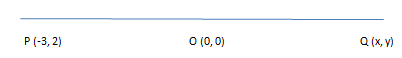QUESTION

# The coordinates of the point P are (-3, 2). Find the coordinates of the point Q which lies on the line joining P and origin such that OP = OQ.

Hint: In this question line formed by two points P and Q and OP = OQ is given. So to find out the coordinate of Q, we will apply the mid-point theorem which states that the x coordinate is the average of the x coordinates of the end points and y coordinate is the average of the y coordinates of the endpoints.We know that if line AB has coordinates as $A({x_1},{y_1})$ and $B({x_2},{y_2})$ then its middle coordinate can be expressed as $\left( {\dfrac{{{x_1} + {x_2}}}{2},\dfrac{{{y_1} + {y_2}}}{2}} \right)$ this is called mid-point theorem.
$\dfrac{{x + \left( { - 3} \right)}}{2} = 0{\text{ and }}\dfrac{{y + 2}}{2} = 0 \\ x = 3{\text{ and y}} = - 2 \\$
Hence the coordinate of $Q(3, - 2)$.